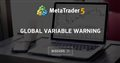# For loop, array & timeframe

Hi guys, don't know if I know what I'm doing, are either lines of code correct?

```   int TimeFrame = {15, 60, 240};

for(i=0; i<ArraySize(TimeFrame); i++)
{
if(TimeFrame[i]==15)
{
return(i);
}
if else(TimeFrame[i]==60)
{
return(i);
}
if else(TimeFrame[i]==240)
{
return(i);
}
}

double BodyOpen = iOpen(pair, TimeFrame[i], 1);```

Or would it be better doing it this way?

```int TimeFrame = {15, 60, 240};

for(i=0; i<ArraySize(TimeFrame); i++)
{
if(TimeFrame[i]==15)
{
return(15);
}
if else(TimeFrame[i]==60)
{
return(60);
}
if else(TimeFrame[i]==240)
{
return(240);
}
}

double BodyOpen = iOpen(pair, TimeFrame[15||60||240], 1);```

There is so much wrong about that code, I do t even know where to start.

What are you trying to accomplish?

Dominik Egert #:
There is so much wrong about that code, I do t even know where to start.

What are you trying to accomplish?

Not surprised. I have many functions such as BodyOpen, which must be calculated separately using the three different timeframes in the array above. The functions must be calculated once a candlestick closes on those given timeframes

Ok, you haven't given details to solve your issue.

`TimeFrame[15||60||240]`

What is this?

```      if(TimeFrame[i]==15)
{
return(15);
}
if else(TimeFrame[i]==60)
{
return(60);
}
if else(TimeFrame[i]==240)
{
return(240);
}```

Dominik Egert #:
Ok, you haven't given details to solve your issue.

Those are the three different timeframes my code needs to execute. In the iOpen function, a timeframe must be given and I thought it was a possible way to implement what I needed. Obviously now I know that wrong but I'm looking a solution to this error

Dominik Egert #:
What is this?

Once again I thought that was a way to implement what I needed

Ok, now write down what you need.

What's the input, what's going to be the output?

Ask yourself. Every function has this principal.
IPO -> Input - Processing - Output

When you design a function, write down what's going to be the input, what's going to be the output.

Then figure out, what information do you need to achieve this output from the given input.

Aquire the data, design data processing.

Now code what you have written. (Use comment-notation for your writings)

Then, to have good code, read examples, debug them step by step. See if you are using the syntax correctly.

Read basics of flow control. (Your if statement and the for loop are hilarious).

Learn about operators. There are multiple types. Boolean, arithmetic, bit arithmetic, pointer arithmetic....

Learn about variable types, memory alignment (pragma pack, ie), casting, converting.

Learn about type attributes (const, static, &, *)

Learn about arrays and their management.

Learn about scope, namespaces, validity, life time, difference about initialization, declaration, definition and assignment.

When you are done with all of that, you may begin to learn OOP. Not before!!

As you go forward, learn about compiler directives, macros and definitions.

As a rule of thumb, if you have to duplicate code, you are probably doing something wrong.

There is so much more to learn, listing it all would be to much and I have left out quite some topics. But this should give you a solid start into what you want to do.

It will take a few days to read all up, and you'll be reading the docs so often until you almost know them by heart.

I wish you lots of success and fun.

I expect you to come back in a few days and tell me what this is actually doing:

`TimeFrame[15||60||240]`

Dominik Egert #:
I expect you to come back in a few days and tell me what this is actually doing:

I am willing to bet he is jsc under a new username.Global variable warning
• 2021.12.23
• www.mql5.com
I am getting the warning "declaration of 'SLPrice' hides global variable". (When I click on the error it takes me to where I've added ***...

Sometimes I ask myself.... No, I don't anymore...

:-)